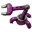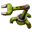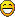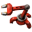## Maximum multiple calculation

Don't know how to use a machine? Looking for efficient setups? Stuck in a mission?
Impatient
Filter InserterPosts: 858
Joined: Sun Mar 20, 2016 2:51 am
Contact:

### Maximum multiple calculation

I have a group of signals with their respective values set in a const combinator. These represent the items required to make 1 of item E. Let's call these signals r1, r2, r3, ... rn. I also have a chest with various items and I am reading its contents. Let's call the signals derived from that c1, c2, c3, ... cn. r- and c-signals with the same index are of the same type (eg "inserter" or "accumulator").

Now I would like to know the maximum amount of E (mE) I can make with the contents of the chest.

Derived just from logic: The maximum is the minimum of the "maximums". The "maximums" are the amounts calculated, when looking at each one required item type separately.

Eg

Code: Select all

``````r1= 2, r2= 3
c1=19, c2=23
q1 = 19/2 = 9
q2 = 23/3 = 7
mE = min(9,7) = 7
``````
My approach so far:
- Step 1: For each pair (rx,cx) I can set up a circuit that performs a division and returns a quotient qx. qx is the whole multiple of rx in cx. The growth rate of combinators for this step is O(n).
- Step 2: I would need to perform a calculation mE = min(q1, q2, q3, ... qn). And I believe the growth rate of combinators in this step would also be O(n). As I would perform m12 = min(q1,q2), m13= min(m12,q3), ... mE = min(m1n-1, qn)

Can you get to mE with growth rates smaller than O(n)?

Last edited by Impatient on Sun Oct 31, 2021 9:12 pm, edited 5 times in total.

mrvn
Smart InserterPosts: 5484
Joined: Mon Sep 05, 2016 9:10 am
Contact:

### Re: Least multiple calculation

Well, the minimum amount you can make out of any chest worth of stuff is 0. I think you mean the maximum.

You can do a binary search for the answer:

Set up a constant combinator (C) with the recipe, both the count for ingredients and negatives for results. If one is a catalyst (input and output) subtract the amount output from the chest with a second constant combinator. Now you want to know the maximum E so that E * r1 < c1, E * r2 < c2, ...

This requires at most 31 steps:

Code: Select all

``````start with E = 0, M = chest content and T = 2^31
as long as T > 0:
if M >= T * C: (subtract and compare EVERYTHING >= 0)
M = M - T * C
E += T
T /= 2
``````
You can implement this as a loop, trading space for time since it needs memory cells then. Or build 31 copies feeding each into the next. The T value could be hardcoded then.

Now a chest is rather small so it's unlikely you can run 2 billion cycles of the recipe from a single chest. Practically you can get away with a lot fewer steps, each step doubles the maximum number of cycles. 10 steps or a maximum of 2047 is probably overkill already.

Note: M will contain the final account, everything that remains of the input and all the output. Except the remaining catalyst needs to be added back. Even works if some extra output is already in the chest.

Tertius
Filter InserterPosts: 465
Joined: Fri Mar 19, 2021 5:58 pm
Contact:

### Re: Maximum multiple calculation

Your challenge is a division, then calculate the maximum of the signals from the result. You cannot do a division of n/m signals with one combinator, the same with calculating a maximum of n signals. It's extremely elaborate to build these operations.

Replace that division by a loop that does subtraktion, and count the number of loop iterations. Start with count=0.
Subtraktion is possible with 1 combinator. Multiply the second operand by -1 with 1 combinator, then use the implicit adding of signals to subtract.
Then look if any of the result is <0 (decider combinator with the Anything operator as input and output)
If there is a result < 0 at the output of that combinator, there is at least 1 source missing for construction, so you have the number of items in the loop counter and are finished.
If there is no result < 0, increment the loop counter and repeat the loop.

Impatient
Filter InserterPosts: 858
Joined: Sun Mar 20, 2016 2:51 am
Contact:

### Re: Least multiple calculation

mrvn wrote:
Sun Oct 31, 2021 3:04 pm
Well, the minimum amount you can make out of any chest worth of stuff is 0. I think you mean the maximum.
Yes, correct. With the previous wording I was referring to the minimum among the quotients, which could have been better. I updated the wording.
Tertius wrote:
Sun Oct 31, 2021 3:35 pm
Your challenge is a division, then calculate the maximum of the signals from the result. ...
Uuuuhm, I am not entirely sure.

Just to be 100% clear: I want the max amount of E, I can make, which is the minimum amongst the results of the divisions (quotients) for each ingredient item type. In other words ... the maximum is the minimum among the "maximums". (' updated the op.)

Impatient
Filter InserterPosts: 858
Joined: Sun Mar 20, 2016 2:51 am
Contact:

### Re: Maximum multiple calculation

I am not happy with the setup of my question. I think, next time I need to factor in the total amount of combinators required and the growth of latency (because of loops) at worst case and maybe some other characteristics which might be important.
Last edited by Impatient on Sun Oct 31, 2021 6:44 pm, edited 1 time in total.

Impatient
Filter InserterPosts: 858
Joined: Sun Mar 20, 2016 2:51 am
Contact:

### Re: Maximum multiple calculation

Tertius wrote:
Sun Oct 31, 2021 3:35 pm
... Replace that division by a loop that does subtraktion, and count the number of loop iterations. ...
Would that mean, in the worst case of ingredient amount = 1 and 2.1 billion items in chest, that algo would loop 2.1 billion times?

Tertius
Filter InserterPosts: 465
Joined: Fri Mar 19, 2021 5:58 pm
Contact:

### Re: Least multiple calculation

Impatient wrote:
Sun Oct 31, 2021 5:47 pm
Just to be 100% clear: I want the max amount of E, I can make, which is the minimum amongst the results of the divisions (quotients) for each ingredient item type. In other words ... the maximum is the minimum among the "maximums". (' updated the op.)
To be honest, I don't really tried to understand what you mean with the minimum of all maximums, because that's too complex for simple Factorio combinator logic.
Impatient wrote:
Sun Oct 31, 2021 2:06 pm
I would like to know the maximum amount of E (mE) I can make with the contents of the chest.
In my own words: You have a chest with ingredients, and you have a const combinator with the ingredient count of some recipe.
You want to know how often you can make the recipe with the contents of the chest.

My first answer stands, and I refine it a bit. It's even easier than I thought. It is a completely different approach to your approach.

In a loop, you add the ingredient count to a temporary sum. In every iteration, you compare the temporary sum with the chest content. If you get one negative signal, you found the number of makes. The loop simulates the extraction of items from the chest. Every iteration, it extracts one set of items and check if one negative signal appears: in this case the loop counter contains the number of possible makes.

Every step is directly possible with 1 combinator operation. The growth rate of combinators is 0 (zero), regardless of the number of ingredients.

In the following description, uppercase letters are inputs or outputs, so they contain many signals. Lowercase letters are single (virtual) signals A, B, C, ... and lowercase words are sums that are created implicitly by adding red and green wire at an input.

let I = ingredient count of all items (the output of the const combinator)
let IN = I * -1. Either you set all negative in the first place or use 1 arithmetic combinator for this.
let IC = contents of the chest

loop outer:
let ingredientsum = IN
let c = 1

loop inner:
; subtract required items from chest content (ingredientsum is negative, so we can use adding)
let diff = ingredientsum + IC

; if any ingredient count becomes negative, we have not enough and need to reset. The number of makes is the previous counter c.
if anything(diff) < 0 then r = 1, else r=0

; terminate inner loop, if need to reset
if r = 1 then goto finished_counting

; the next 2 calculations use the regular increase mechanism for counters (loopback decider combinator)
let c = c + 1
let ingredientsum = ingredientsum + IN
goto inner

finished_counting:
save c from previous tick in a memory cell m. This is our number of makes.
; repeat
goto outer

Currently, I'm not yet sure how to implement the memory cell. Its input are r and c, and it needs to store value c to m if r=1, and keep value m memorized if r=0.

The 2 loops can be accomplished with 2 combinators. 1 combinator for extracting the counter c. The number of combinators for the memory cell isn't clear, I found 3 combinators for that kind of task, which means a complete solution can be made with as many as 6 combinators, with an arbitrary number of recipe ingredients (as far as I remember, there are never more than 6 anyway, which is the satellite).

The latency is the number of iterations required until the diff becomes negative. Can be quite long for simple recipes. But while the calculation runs, you read the value from the memory cell, so that's probably not that important.

Tertius
Filter InserterPosts: 465
Joined: Fri Mar 19, 2021 5:58 pm
Contact:

### Re: Maximum multiple calculation

Impatient wrote:
Sun Oct 31, 2021 6:50 pm
Tertius wrote:
Sun Oct 31, 2021 3:35 pm
... Replace that division by a loop that does subtraktion, and count the number of loop iterations. ...
Would that mean, in the worst case of ingredient amount = 1 and 2.1 billion items in chest, that algo would loop 2.1 billion times?
Yes. Hmm. A chest usually contains much less items, a few thousand, and you will probably not use that logic for simple ore smelting, but for some more complex recipes. If you use it for ore smelting = 4800 items, 4800 iterations = 4800 / 60 ticks = 80 seconds. If you use it for more complex recipes which use more ingredients and items with smaller stack sizes, it will use significantly less time. A few seconds at most, I would say.

mrvn
Smart InserterPosts: 5484
Joined: Mon Sep 05, 2016 9:10 am
Contact:

### Re: Maximum multiple calculation

Tertius wrote:
Sun Oct 31, 2021 7:37 pm
Impatient wrote:
Sun Oct 31, 2021 6:50 pm
Tertius wrote:
Sun Oct 31, 2021 3:35 pm
... Replace that division by a loop that does subtraktion, and count the number of loop iterations. ...
Would that mean, in the worst case of ingredient amount = 1 and 2.1 billion items in chest, that algo would loop 2.1 billion times?
Yes. Hmm. A chest usually contains much less items, a few thousand, and you will probably not use that logic for simple ore smelting, but for some more complex recipes. If you use it for ore smelting = 4800 items, 4800 iterations = 4800 / 60 ticks = 80 seconds. If you use it for more complex recipes which use more ingredients and items with smaller stack sizes, it will use significantly less time. A few seconds at most, I would say.
And doing a binary search will take 60-120 ticks for any input, less if you limit it to chest size.

mrvn
Smart InserterPosts: 5484
Joined: Mon Sep 05, 2016 9:10 am
Contact:

### Re: Least multiple calculation

Impatient wrote:
Sun Oct 31, 2021 5:47 pm
Tertius wrote:
Sun Oct 31, 2021 3:35 pm
Your challenge is a division, then calculate the maximum of the signals from the result. ...
Uuuuhm, I am not entirely sure.

Just to be 100% clear: I want the max amount of E, I can make, which is the minimum amongst the results of the divisions (quotients) for each ingredient item type. In other words ... the maximum is the minimum among the "maximums". (' updated the op.)
The problem he is referring too is that you can not easily compute "red wire / green wire". You would need one combinator for every q1, q2, ..., qn. But you can add a bunch of signals in a single combinator or even multiply 2 wires with iirc 5 combinators. So whenever you have a division of many signals try hard to see if you can modify that somehow to use a multiplication instead.

Impatient
Filter InserterPosts: 858
Joined: Sun Mar 20, 2016 2:51 am
Contact:

### Re: Least multiple calculation

Tertius wrote:
Sun Oct 31, 2021 7:06 pm
... It's even easier than I thought. It is a completely different approach to your approach. ...
My approach is the beginners approach, doing everything for each signal separately.Last edited by Impatient on Sun Oct 31, 2021 9:19 pm, edited 1 time in total.

Impatient
Filter InserterPosts: 858
Joined: Sun Mar 20, 2016 2:51 am
Contact:

### Re: Least multiple calculation

mrvn wrote:
Sun Oct 31, 2021 3:04 pm
...
This requires at most 31 steps:

Code: Select all

``````start with E = 0, M = chest content and T = 2^31
as long as T > 0:
if M >= T * C: (subtract and compare EVERYTHING >= 0)
M = M - T * C
E += T
T /= 2
``````
...
That is for getting the quotients only, right? Or does

Code: Select all

``````(subtract and compare EVERYTHING >= 0)
``````
include getting the smallest quotient?

mrvn
Smart InserterPosts: 5484
Joined: Mon Sep 05, 2016 9:10 am
Contact:

### Re: Least multiple calculation

Impatient wrote:
Sun Oct 31, 2021 9:17 pm
mrvn wrote:
Sun Oct 31, 2021 3:04 pm
...
This requires at most 31 steps:

Code: Select all

``````start with E = 0, M = chest content and T = 2^31
as long as T > 0:
if M >= T * C: (subtract and compare EVERYTHING >= 0)
M = M - T * C
E += T
T /= 2
``````
...
That is for getting the quotients only, right? Or does

Code: Select all

``````(subtract and compare EVERYTHING >= 0)
``````
include getting the smallest quotient?

It checks if it can build 1 billion. Then with the rest it checks if it can build 512 million. Again with the rest it checks if it can build 256 million, then 128, 64, 32, ... At the end it will have figured out the total anywhere between 0 and 2 billion.

farcast
Long Handed InserterPosts: 72
Joined: Fri Jul 06, 2018 8:25 am
Contact:

### Re: Maximum multiple calculation

It's possible to use pairwise multiplication to perform pairwise division, so that a separate circuit is needed per recipe instead of per ingredient. Divide some large number P by each recipe value (divide by each is a new feature), pairwise multiply the results with each chest value, then divide away the factor of P. From there, you just need to feed the results into a minimum value circuit to get the maximum items that can be crafted.Max Craft Calculator.jpg (173.47 KiB) Viewed 3295 times
On the left is the pairwise division circuit, on the right is the minimum value finder. The decider at the end of the division circuit is just to ensure every signal is always present so that the minimum value finder correctly outputs zero if one of the ingredients is missing. The minimum value finder holds the last result at the output until the new result has been stable for 3 ticks.

The minimum value circuit works by calculating the average value of the inputs, then a new average with the inputs that are less than or equal to the previous average. Repeat until all inputs still used have the same value, then the average is the minimum value. In the case where all inputs are greater than the average, the average is pinned to int max. Some finagling was done to prevent the average signal from being included in it's own calculation, and the constant combinator with a -1 at the output is to compensate for the +1 on all the inputs to ensure they are always present.

The calculation time from a change in the chest value to the corresponding change in output value is 10 ticks minimum + 2 ticks for every loop needed to find the minimum value. Worst case is 1 loop per ingredient, best case is no extra loop time.

It should give accurate results as long as the P value is set to a common multiple of the recipe values.

Edit: I simplified the reset condition for the min value finder as it was needlessly complicated. A purely aesthetic change.
More edit: Added an explanation for the minimum value circuit, and re-wired the input of the division circuit so that both inputs have the same calculation time as mentioned above.

Max Craft Calculator
Efficient inefficient design.